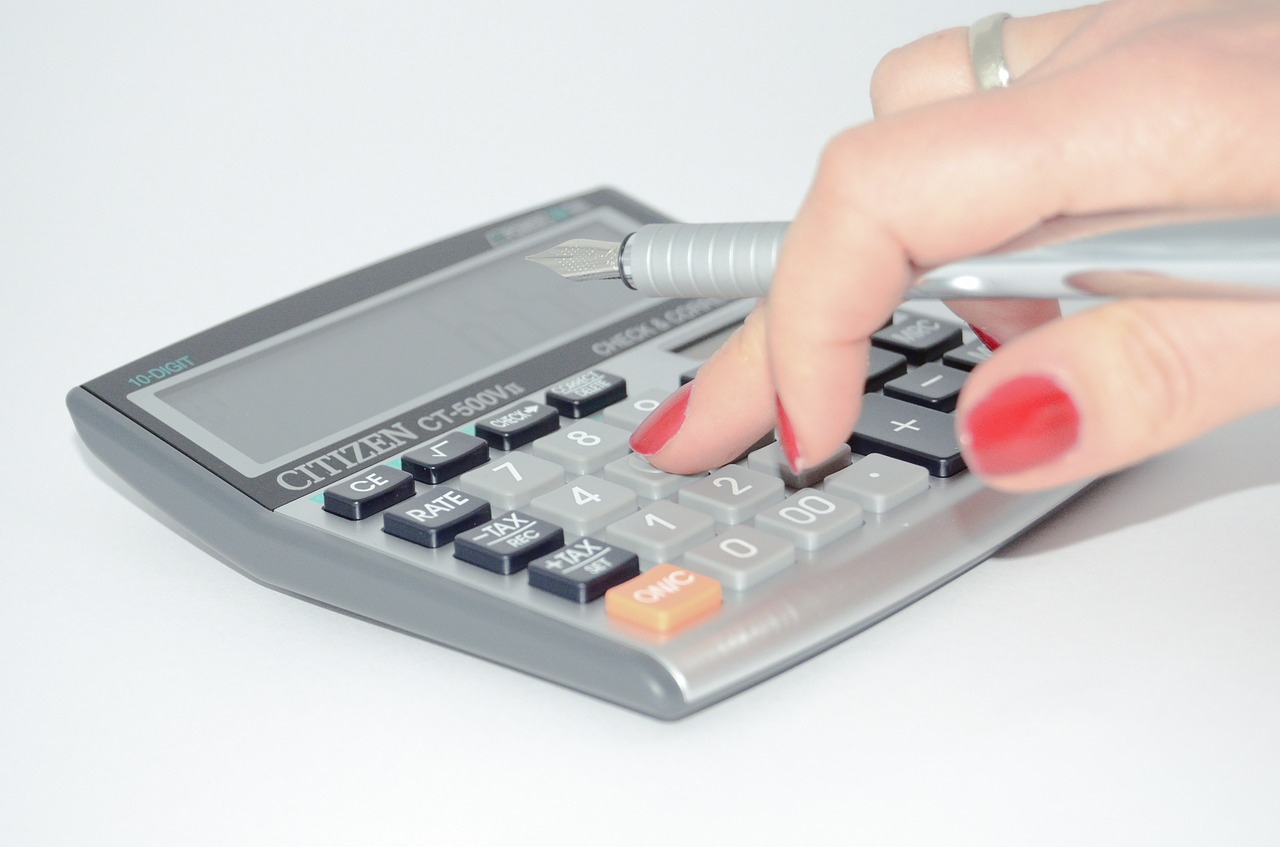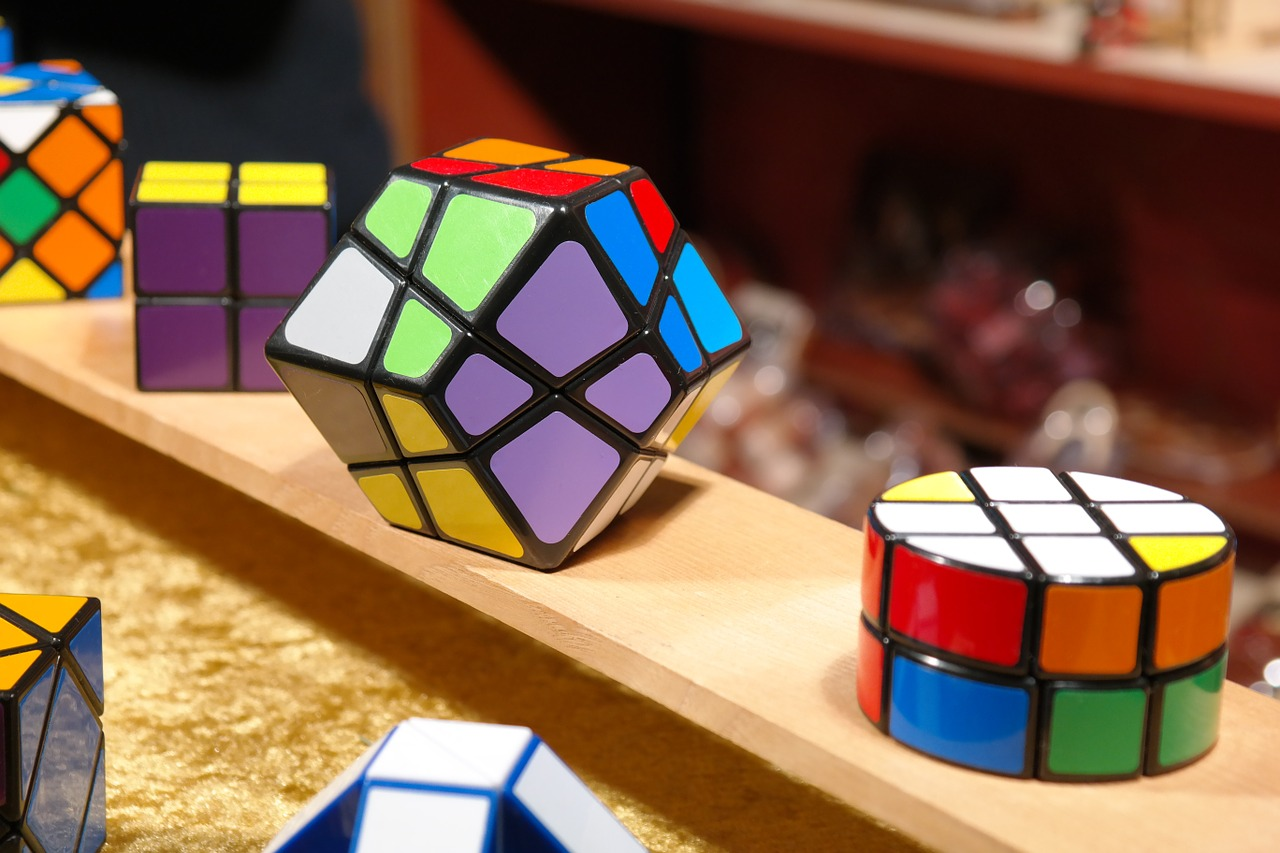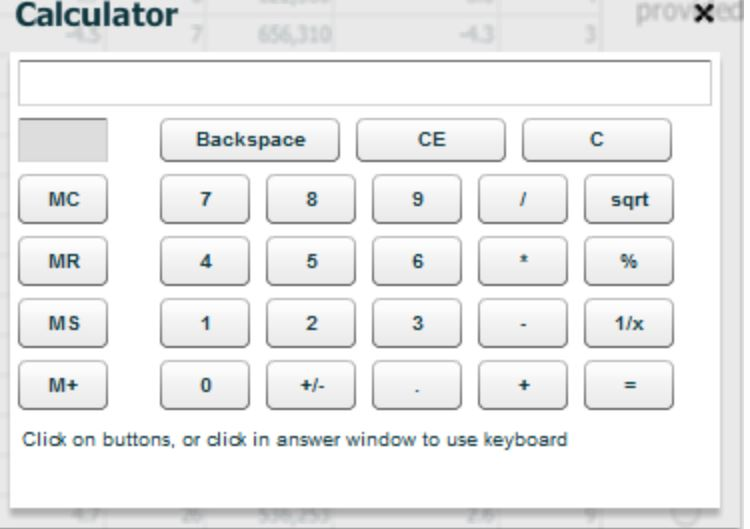# GMAT Calculator: What Is It? When Can You Use It?Can you use a calculator on the GMAT? Yes, but probably not as much as you’d like.

In this article, I’ll ago over which sections of the GMAT have an onscreen calculator, the functions of the GMAT calculator, and how to use it most effectively on test day.

## Is There a GMAT Calculator?

So, can you use a calculator on the GMAT? The short answer is sometimes.

There is an onscreen calculator on the Integrated Reasoning (IR) section, but there is no calculator on the Quantitative (Quant) section. On that section, you’ll have to do all your calculations by hand.Don’t worry! You can succeed on the Quant section without a calculator.

Not sure how or what to study? Confused by how to improve your score in the shortest time possible? We've created the only Online GMAT Prep Program that identifies your strengths and weaknesses, customizes a study plan, coaches you through lessons and quizzes, and adapts your study plan as you improve.

We believe PrepScholar GMAT is the best GMAT prep program available, especially if you find it hard to organize your study schedule and don't want to spend a ton of money on the other companies' one-size-fits-all study plans.## No GMAT Calculator on the Quant Section?!

If that last sentence scared you, don’t panic. There are several reasons not to worry:

1. The GMAT Quantitative section is not designed to test you on complicated mathematical skills or specific calculations. Instead, the Quant section draws from secondary-level math skills like basic algebra and geometry. In fact, the majority of Quant questions can be answered without any calculations beyond estimation.
2. You will have a notepad with plenty of space provided to you by the proctor on test day. If you absolutely need to do some calculations, you can complete them manually there.
3. Even if you feel uncomfortable doing arithmetic without a GMAT calculator, it won’t take long for you to improve with practice.

Working without a calculator may take some getting used to, but it’s not as scary as it sounds.

## How to Succeed Without a Calculator in the GMAT Quant Section

While you shouldn’t be overly concerned about not having a calculator during the Quant section, there are ways to prepare effectively so you can do your best come exam day.

### #1: Practice, Practice, Practice

If you don’t feel comfortable doing arithmetic quickly in your head, you should start practicing now. Of course, you should complete all your Quant practice tests without a calculator, but you can also add, multiply, subtract, and divide when you pay bills, check out at the grocery store, or calculate a tip. Try to use a calculator as rarely as you can in preparation for the GMAT.

### #2: Estimate

The key to completing many Quant questions efficiently is estimation. You should always try to round up or down to find more manageable, less unwieldy numbers (often ones that end in zero or 5) for the purpose of calculations. If a problem or answer choice requires you to multiply 44.6 by 11.15, for example, you can multiply 45 by 10 instead and still end up with the correct answer.

### #3: Use Halving and Doubling to Simplify Calculations

Another useful skill when completing the Quant section without a GMAT calculator is called ‘halving and doubling,’ which doubles (or otherwise multiplies) one number in a calculation and halves (or otherwise divides by the same factor) the other to result in a friendlier pair of numbers for someone without a calculator. Let’s take 25 times 24, for example. Divide 24 by 6 to get 4–easy enough–and multiple 25 by 6 to get 150. What you’re left with is 4 times 150, a much easier calculation (600).Developing your logic skills is important to prepare for the Quant and Integrated Reasoning sections.

## GMAT Calculator: What It Does and How to Use It

Unlike the Quant section, the Integrated Reasoning (IR) section does have an onscreen calculator available. You’ll simply click on “Calculator” at the top lefthand corner of the screen, and the calculator will appear in the middle:As you can see, the GMAT calculator has standard basic functions. The fairly self-explanatory top row of the calculator consists of Backspace (which clears only the last digit you typed), CE (clear entry, or what you entered in the line), and C (clear all, or everything that is stored in previous operations).

The right column of the IR calculator contains a few other basic functions. The ‘sqrt’ (square root) button will calculate the square root of any number you just typed.

The % percentage button will help you if you want to increase or decrease a number by a certain percentage: for example, typing 100 + 10% will result in 110.

The ‘1/x’ button calculates the reciprocal of the entry currently on the screen. For example, typing “5” and then the 1/x button will show you .2, which is the decimal equivalent of ⅕.

The left column consists of the ‘memory’ functions. These are especially helpful for calculations that require several steps.

1. MS (memory store) stores the current entry in the calculator’s memory for subsequent use.
2. MR (memory recall) displays the latest number stored in memory on the screen so you can use it for the next step of your calculation.
3. M+ (memory addition) adds the current entry to whatever is in the current memory. This button is helpful when you need to add a long series of numbers and don’t want to retype each one.
4. Finally, MC (memory clear) erases whatever is in the current memory. You should click this button before every new calculation scenario.

While the IR GMAT calculator can be a helpful tool, you don’t want to use it too often or you’ll waste more time than you save. You can use many of the same tips and tricks on the IR section that you’ll use on the Quant section, where you don’t have a calculator. The same skills you practice to prepare for the Quant section will also help you on the IR section.

## Review: Can You Use a Calculator on the GMAT?

There is no calculator available on the Quantitative section of the GMAT, but there is one on the Integrated Reasoning. The GMAT calculator has the same functions as a standard four-function calculator with square roots.

Though the idea of taking a math test with no calculator can be intimidating, you shouldn’t panic. The GMAT Quant section doesn’t test your ability to do complex arithmetic — you’ll only need to do basic calculations and can rely on estimation for anything more complicated.## What’s Next?

For more information on how to make effective use of the scratch pad you’ll be provided with during the GMAT, read our article on GMAT scratch paper.

Wondering what topics you need to review before trying to master the Quant section? Our guide to the math you need for the GMAT can help.

Want to improve your GMAT score by 60 points?

We have the industry's leading GMAT prep program. Built by Harvard, MIT, Stanford, and Wharton alumni and GMAT 99th percentile scorers, the program learns your strengths and weaknesses and customizes a curriculum so you get the most effective prep possible.To delve more deeply into GMAT Math strategies, check out our guide to mastering the Quant section.